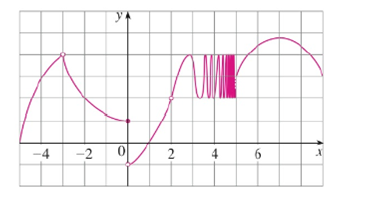Chapter 2.2, Problem 6E

Chapter
Section
Textbook Problem

For the function h whose graph is given, state the value of each quantity, if it exists. If it does not exist, explain why.(a) lim x → 3 − h ( x ) (b) lim x → 3 + h ( x ) (c) lim x → − 3 h ( x ) (d) h ( − 3 ) (e) lim x → 0 − h ( x ) (f) lim x → 0 + h ( x ) (g) lim x → 0 h ( x ) (h) h ( 0 ) (i) lim x → 2 h ( x ) (j) h ( 2 ) (k) lim x → 5 + h ( x ) (l) lim x → 5 − h ( x )(a)

To determine

To find: The value of limx3h(x).

Explanation

From the given graph, it is found that the curve move towards y=4 as x approaches −3 from the left, that is x>3

(b)

To determine

To find: The value of limx3+h(x).

(c)

To determine

To find: The value of limx3h(x).

(d)

To determine

To find: The value of h(3).

(e)

To determine

To find: The value of limx0h(x).

(f)

To determine

To find: The value of limx0+h(x).

(g)

To determine

To find: The value of limx0h(x).

(h)

To determine

To find: The value of h(0).

(i)

To determine

To find: The value of limx2h(x).

(j)

To determine

To find: The value of h(2).

(k)

To determine

To find: The value of limx5+h(x).

(l)

To determine

To find: The value of limx5h(x).

Still sussing out bartleby?

Check out a sample textbook solution.

See a sample solution

The Solution to Your Study Problems

Bartleby provides explanations to thousands of textbook problems written by our experts, many with advanced degrees!

Get Started

Find more solutions based on key concepts